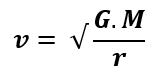Satellites in Circular Orbits - العلم نور

# العلم نور

{ وَقُلْ رَبِّ زِدْنِي عِلْمًا }

## الجمعة، 20 سبتمبر 2019

Satellites in Circular OrbitsSatellites in Circular Orbits

Satellites
Satellites refer to the objects that orbits the earth or any massive body. They can be natural like the moon to the Earth or man-made like satellites that orbit our planet which help in taking some pictures for it or for other planets to provide some information about the universe.

There are some physical principles that governs all types of satellites either they are natural satellites or man-made satellites, mathematical equations are used for describing these physical principles for satellites. We have to understand that satellites are projectiles as they move in their orbits freely under the effect of gravity only. The orbits of satellites are circular and their motion is governed by the same physical laws of circular motion.

Satellites in Circular Orbits
Circular orbit is the orbit which has the shape of circle, this circle has a constant radius when the body orbits around any massive body and is affected by the gravitational force of this body. In this type of orbits, there are two objects exist. The larger one will be the center of the circle which will be fixed and the smaller one will move around it in a circular motion.

For example, when a satellite orbits the Earth, the Earth is fixed and the satellite move around it in circular orbit and the only force act on the satellite will be the gravitational force of the Earth. There are some principles of satellites in circular motion, these principles will be discussed below in the next section.

The Principles of Satellites in Circular Orbits
The principles of satellites in circular orbit will be the same of circular motion principles. The velocity, acceleration and force of orbiting satellites will be described as illustrated above in the graph.

For velocity vector, it will be always tangent to the circle of satellite’s orbit at each point on the path of motion. This velocity can be calculated mathematically according to the following equation:velocity vector
Where,
-        v: is the velocity of the satellite around the planet.
-        G: is the gravitational constant, it equals 6.674 × 10−11 m3kg−1s−2.
-        M: is the mass of the planet (larger object).
-        r: is the distance between the centre of the planet and satellite.
For acceleration vector, its direction is perpendicular to the velocity vector, and its effect will be towards the centre of the orbit. This acceleration source will be the net force which acts inwards in the same direction which is the direction of gravity action.
For force vector, the gravity of the planet will generate a centripetal force, this force is important for the satellite to continue moving in the same direction and with the same speed. This gravitational force between the satellite and the planet or between any two massive bodies will be calculated mathematically by the following equation:
-        G: is the gravitational constant, it equals 6.674 × 10−11 m3kg−1s−2.
-        M: is the mass of the planet (larger object).
-        r: is the distance between the center of the planet and satellite.
-        m: is the mass of the satellite.